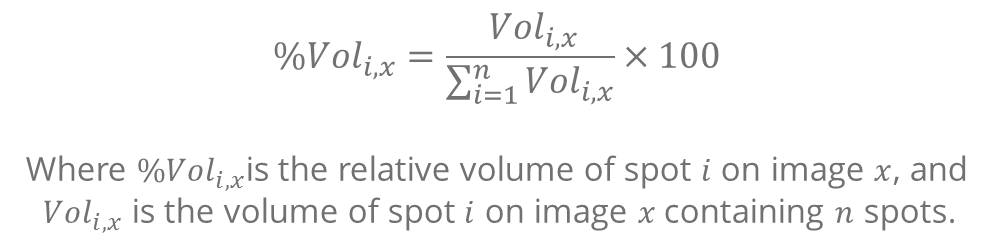In the detection step, spots are detected on a fusion image (which is not displayed, but which is generated based on the images marked for inclusion). The resulting spot pattern is propagated to the individual images, taking into account the deformation calculated in the alignment.

For each spot on an image, MelanieTM  then computes the following quantification measures, based on the calibrated pixel gray values:

• Area: The area of a spot is the surface delimited by the spot border, and is expressed in mm2. When looking at the same spot on different images, you will observe that the areas vary a little. This is because the spot shapes are warped proportionally to the deformation calculated in the alignment, when the spots are propagated from the fusion image to each individual image.
• Intensity: The intensity of a spot is based on the highest gray values in the spot from which the background has been withdrawn. The background is defined as the minimum gray value on the spot border.
• Vol: The non-normalized volume of a spot is the sum of the background-subtracted gray values of all pixels delimited by the spot border. The background is defined as the minimum gray value on the spot border. However, when normalization is applied, the Vol field will always display the normalized volume. Learn more about how normalized volumes are calculated.
• Vol Ratio: Volume ratios are only calculated for DIGE experiments. They indicate the change in spot volume between the spot in the current image and the spot in the DIGE reference image.
• %Vol: The relative volume of a spot is the volume of the spot normalized to the total volume over all the spots in the image. It is calculated using the formula below.